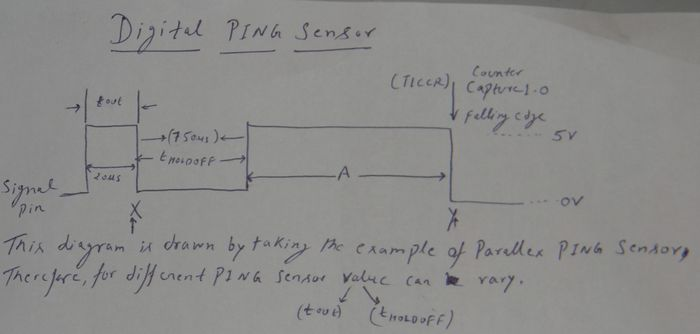# PING))) Ultrasonic Sensor

## Overview

The PING))) Ultrasonic works by sending sound waves and then the sensor signal emits a PWM that needs to be timed to detect the distance. The PWM width depends on the time it takes for the sound waves to bounce back from an object.

## Interface Details

The interface of this sensor is simple, just Power, Ground, and PWM Signal, but this signal wire is multiplexed between input and output. Here are the steps needed:

• Connect to your CPU Pin, such as P0.X
• Configure P0.X as output, and give a 20uS Pulse of HIGH then LOW
• Immediately configure P0.X as input
• Take a snapshot of a hardware timer or start a hardware timer
• Enable P0.X as falling edge interrupt
• --> After some time <--
• P0.X will go HIGH, and then LOW and the width of this HIGH wave corresponds to the object distance
• Interrupt on falling edge will now trigger, so compute the delta of the hardware timer
• Disable falling edge interrupt otherwise when you trigger this sensor again (bullet 2 above), the interrupt will fire unintentionally.

## LPC2148 FreeRTOS Hints

• Since FreeRTOS uses Timer0, simply use Timer1
• The FreeRTOS sample code provided already starts TIMER1, so:
• When you want to compute the delta, save the timer value when you trigger the PING))) Sensor by:
`unsigned int timerValueAtTrigger = portGET_RUN_TIME_COUNTER_VALUE();`
• When the ISR occurs, compute the delta:
`unsigned int timerValueAtIsr = portGET_RUN_TIME_COUNTER_VALUE();`
`unsigned int timerDelta = (timerValueAtIsr - timerValueAtTrigger);`
• At TIMER1_PC=32 (default), each tick = 1/(48Mhz/32) = 666ns, so:
`unsigned int deltaInMicroSec = timerDelta * 0.666;`
• ``` Now use the PING))) datasheet to convert Micro-seconds to inches.```
``` Timer/Capture Based PING sensor: Things need to be done before start interfacing PING sensor with LPC2148: 1. Read chapter 15, Timer/Counter, from the datasheet of LPC2148. 2. Make sure the PING sensor is digital one. 3. Make sure do not use TIMER0 because it is used by FreeRTOS. 4. Select pin of LPC2148 that can be set for Capture 1, for example Pin 10. Pin description of PING sensor There are three pins in digital PING sensor. a) 5v pin b) Signal Pin c) Ground Points to be noted: 1. FreeRTOS starts TIMER1 and TIMER0 by itself. 2. OR you can also initiate TIMER1 by yourself. 3. OR you can set only T1CCR register (Atleast). How to Write TIMER1 driver? 1. First enable the timer reset by setting bit 1 as ‘1’ in TITCR. 2. Then set the Prescale Register (T1PR) according to number of PCLK you want to count before incrementing Timer Counter (T1TC). Once TITC increments T1PC (Prescale Counter Register) is reset. You can set T1PR to zero so that T1TC will increment on every PCLK. 3. The third register need to set is Capture Control Register (T1CCR). This register can be set for one of the following functions: a. Capture on Rising edge b. Capture on Falling edge c. Interrupt on Capture pin CAPn.0 4. Finally, enable the TIMER by enabling bit 0 in T1TCR Working of PING sensor In order to activate the sensor, the host microcontroller (LPC2148) will send a short pulse of duration 20 microseconds on signal pin. Just after the pulse will end, set the signal pin as input. Then, at that moment take the snapshot of the PCLK through T1TC register. Snapshot means note down the time at point X. Initialize the register T1CCR so that when falling edge will come then T1CCR register store the time in T1TC register, which will be at point Y. Now, subtract point X from Y. Find out the HOLDOFF time from the datasheet of PING sensor. Mostly, it is around 750us. HOLDOFF is the time between the PING sensor receives activation signal from host microcontroller and PING sensor emits ultrasonic wave to detect object. Suppose, you get Z by subtracting X from Y. Then, subtract 750us from Z. Z = Y – X A = Z – 750us A’ is the time taken by wave to go and come back; therefore the one side distance will be ‘A’/2 . B = A/2 Now, divide ‘B’ by 73.746 to get the distance in inches. The value 73.746 is taken according to the Parallax digital PING sensor. It could vary for another PING sensor; therefore, please check the datasheet of your PING sensor.Figure 1: Working of Ping Sensor```